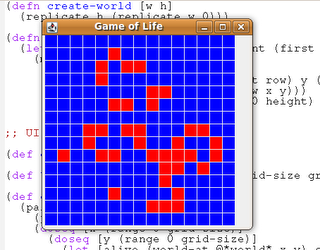## Monday, 5 January 2009

### The Game of Life in Clojure.Conway's Game of Life is by far the most famous example of cellular automaton. It's not really a game, you set an initial state and then watch it evolve.

The "game" is played on a grid with each cell having two states (alive or dead). The next state can be computed from the previous one using the following rules:

1. Any live cell with fewer than two live neighbours dies, as if by needs caused by under-population.
2. Any live cell with more than three live neighbours dies, as if by overcrowding.
3. Any live cell with two or three live neighbours lives, unchanged, to the next generation.
4. Any tile with exactly three live neighbours cells will be populated with a living cell.

The first thing we need to do is determine a representation for a grid. I've gone for a simple approach of representing a grid as a list of lists. `create-world` creates a blank grid

`(defn create-world [w h]  (replicate h (replicate w 0)))`

Now we need some helper functions for getting the state of an individual element and toggling values.

`(defn world-at [world x y]  (if (and (>= x 0) (>= y 0) (< x (count world)) (< y (count (first world))))    (nth (nth world x) y)    0))(defn toggle [x]  (if (= x 0) 1 0))(defn toggle-row-at [row pos]  (map (fn [x] (if (= pos (first x)) (toggle (second x)) (second x))) (zipmap (range 0 (count row)) row)))(defn toggle-pos [world x y]  (map (fn [v] (if (= (first v) x)      (toggle-row-at (second v) y)            (second v)))        (zipmap (range 0 (count world)) world)))`

`toggle-row-at` and `toggle-pos` both feel wrong to me - I'm not sure of a better way to carry position information into a map function (for example, I want to perform a map operation with positional information - zip is the only way I can see of doing that - wonder if there is a better way?).

Armed with functions to explore the world we need to write the logic to transition from one-state to the next.

`(defn neighbour-count [world x y]  (+ (world-at world (dec x) (dec y)) (world-at world x (dec y)) (world-at world (inc x) (dec y))     (world-at world (dec x) y) (world-at world (inc x) y)     (world-at world (dec x) (inc y)) (world-at world x (inc y)) (world-at world (inc x) (inc y))))(defn new-state [world x y]  (let [neighbours (neighbour-count world x y) alive (world-at world x y)]    (cond      (and (= alive 1) (< neighbours 2)) 0 ;; under population     (and (= alive 1) (> neighbours 3)) 0 ;; over-crowding     (and (= alive 1) (or (= 2 neighbours) (= 3 neighbours))) 1 ;; unchanged to the next generation     (and (= 3 neighbours)) 1 ;; any tile with exactly 3 live neighbour cells becomes alive     :else 0)))`

`new-state` encodes the rules, and `neighbour-count` just does exactly what it says on the tin. Now all we need to do is apply `new-state` to each cell in the grid and produce the next one. This is suprisingly simple:

`(defn life-step [w]  (let [width (count w) height (count (first w))]    (map      (fn [row] (map (fn [col]         (let [x (first row) y (first col)]   (new-state w x y)))      (zipmap (range 0 height) (second row))))     (zipmap (range 0 width) w))))`

It again makes use of the `zipmap` idiom for applying mapping function with positional information. I wish I could find a reference which states whether this is a good thing or a bad thing :)

In order to implement the GUI around this, we define `*world*` as an atom, and provide a mouse listener which on a left-click toggles elements, and on a right-click moves forward one step. Animating it would be fairly simple too (just hook up a Swing timer a la bubble sort).

Full code is availabe on my Git Repository

1.2.